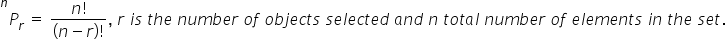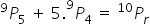Maths-
General
Easy

###Hint:

Here we need to find the value of the given variable. Here we will first apply the formula of the permutation to simplify the equation. Then we will apply the mathematical operations that will be required to simplify the given expression further. After simplifying the terms, we will get the value of the required variable.
Formula used:#### Detailed Solution Here we need to find the value of the given variable.The given expression is :## Book A Free Demo+91